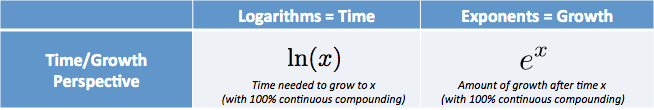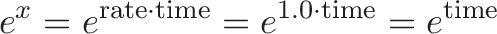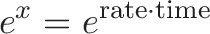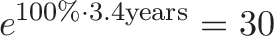# Demystifying the Natural Logarithm (ln)

After understanding the exponential function, our next target is the natural logarithm.

Given how the natural log is described in math books, there’s little “natural” about it: it’s defined as the inverse of $e^x$, a strange enough exponent already.

But there’s a fresh, intuitive explanation: The natural log gives you the time needed to reach a certain level of growth.Suppose you have an investment in gummy bears (who doesn’t?) with an interest rate of 100% per year, growing continuously. If you want 10x growth, assuming continuous compounding, you’d wait only $\ln(10)$ or 2.302 years. Don’t see why it only takes a few years to get 10x growth? Don’t see why the pattern is not 1, 2, 4, 8? Read more about e.

e and the Natural Log are twins:

• $e^x$ is the amount we have after starting at 1.0 and growing continuously for $x$ units of time
• $\ln(x)$ (Natural Logarithm) is the time to reach amount $x$, assuming we grew continuously from 1.0

Not too bad, right? While the mathematicians scramble to give you the long, technical explanation, let’s dive into the intuitive one.

The number e is about continuous growth. As we saw last time, $e^x$ lets us merge rate and time: 3 years at 100% growth is the same as 1 year at 300% growth, when continuously compounded.

We can take any combination of rate and time (50% for 4 years) and convert the rate to 100% for convenience (giving us 100% for 2 years). By converting to a rate of 100%, we only have to think about the time component:$\displaystyle{e^x = e^{\text{rate} \cdot \text{time}} = e^{1.0 \cdot \text{time}} = e^{\text{time}}}$

Intuitively, $e^x$ means:

• How much growth do I get after after x units of time (and 100% continuous growth)
• For example: after 3 time periods I have $e^3$ = 20.08 times the amount of “stuff”.

$e^x$ is a scaling factor, showing us how much growth we’d get after $x$ units of time.

## Natural Log is About Time

The natural log is the inverse of $e^x$, a fancy term for opposite. Speaking of fancy, the Latin name is logarithmus naturali, giving the abbreviation ln.

Now what does this inverse or opposite stuff mean?

• $e^x$ lets us plug in time and get growth.
• $\ln(x)$ lets us plug in growth and get the time it would take.

For example:

• $e^3$ is 20.08. After 3 units of time, we end up with 20.08 times what we started with.
• $\ln(20.08)$ is about 3. If we want growth of 20.08, we’d wait 3 units of time (again, assuming a 100% continuous growth rate).

With me? The natural log gives us the time needed to hit our desired growth.

## Logarithmic Arithmetic Is Not Normal

You’ve studied logs before, and they were strange beasts. How’d they turn multiplication into addition? Division into subtraction? Let’s see.

What is $\ln(1)$? Intuitively, the question is: How long do I wait to get 1x my current amount?

Zero. Zip. Nada. You’re already at 1x your current amount! It doesn’t take any time to grow from 1 to 1.

• $\ln(1) = 0$

Ok, how about a fractional value? How long to get 1/2 my current amount? Assuming you are growing continuously at 100%, we know that $\ln(2)$ is the amount of time to double. If we reverse it (i.e., take the negative time) we’d have half of our current value.

• $\ln(.5) = – \ln(2) = -.693$

Makes sense, right? If we go backwards .693 units (negative seconds, let's say) we’d have half our current amount. In general, you can flip the fraction and take the negative: $\ln(1/3) = – \ln(3) = -1.09$. This means if we go back 1.09 units of time, we’d have a third of what we have now.

Ok, how about the natural log of a negative number? How much time does it take to “grow” your bacteria colony from 1 to -3?

It’s impossible! You can’t have a “negative” amount of bacteria, can you? At most (er… least) you can have zero, but there’s no way to have a negative amount of the little critters. Negative bacteria just doesn’t make sense.

• $\ln(\text{negative number}) = \text{undefined}$

Undefined just means “there is no amount of time you can wait” to get a negative amount. (Well, if we use imaginary exponentials, there is a solution. But today let's keep it real.)

## Logarithmic Multiplication is Mighty Fun

How long does it take to grow 9x your current amount? Sure, we could just use ln(9). But that’s too easy, let’s be different.

We can consider 9x growth as tripling (taking $\ln(3)$ units of time) and then tripling again (taking another $\ln(3)$ units of time):

• Time to grow 9x = $\ln(9)$ = Time to triple and triple again = $\ln(3) + \ln(3)$

Interesting. Any growth number, like 20, can be considered 2x growth followed by 10x growth. Or 4x growth followed by 5x growth. Or 3x growth followed by 6.666x growth. See the pattern?

• $\ln(a*b) = \ln(a) + \ln(b)$

The log of a times b = log(a) + log(b). This relationship makes sense when you think in terms of time to grow.

If we want to grow 30x, we can wait $\ln(30)$ all at once, or simply wait $\ln(3)$, to triple, then wait $\ln(10)$, to grow 10x again. The net effect is the same, so the net time should be the same too (and it is).

How about division? $\ln(5/3)$ means: How long does it take to grow 5 times and then take 1/3 of that?

Well, growing 5 times is $\ln(5)$. Growing 1/3 is $-\ln(3)$ units of time. So

• $\ln(5/3) = \ln(5) – \ln(3)$

Which says: Grow 5 times and “go back in time” until you have a third of that amount, so you’re left with 5/3 growth. In general we have

• $\ln(a/b) = \ln(a) – \ln(b)$

I hope the strange math of logarithms is starting to make sense: multiplication of growth becomes addition of time, division of growth becomes subtraction of time. Don’t memorize the rules, understand them.

## Using Natural Logs With Any Rate

“Sure,” you say, “This log stuff works for 100% growth but what about the 5% I normally get?”

It’s no problem. The “time” we get back from $\ln()$ is actually a combination of rate and time, the “x” from our $e^x$ equation. We just assume 100% to make it simple, but we can use other numbers.

Suppose we want 30x growth: plug in $\ln(30)$ and get 3.4. This means:

• $e^x = \text{growth}$
• $e^{3.4} = 30$

And intuitively this equation means “100% return for 3.4 years is 30x growth”. We can consider the equation to be:$\displaystyle{e^{x} = e^{\text{rate} \cdot \text{time}}}$$\displaystyle{e^{100 \% \cdot 3.4 \text{years}} = 30}$

We can modify “rate” and “time”, as long as rate * time = 3.4. For example, suppose we want 30x growth — how long do we wait assuming 5% return?

• $\ln(30) = 3.4$
• $\text{rate} * \text{time} = 3.4$
• $.05 * \text{time} = 3.4$
• $\text{time} = 3.4 / .05 = 68 \text{years}$

Intuitively, I think "$\ln(30) = 3.4$, so at 100% growth it will take 3.4 years. If I double the rate of growth, I halve the time needed."

• 100% for 3.4 years = 1.0 * 3.4 = 3.4
• 200% for 1.7 years = 2.0 * 1.7 = 3.4 [200% growth means half the time]
• 50% for 6.8 years = 0.5 * 6.8 = 3.4 [50% growth means double the time]
• 5% for 68 years = .05 * 68 = 3.4 [5% growth means 20x the time]

Cool, eh? The natural log can be used with any interest rate or time as long as their product is the same. You can wiggle the variables all you want.

## Awesome example: The Rule of 72

The Rule of 72 is a mental math shortcut to estimate the time needed to double your money. We’re going to derive it (yay!) and even better, we’re going to understand it intuitively.

How long does it take to double your money at 100% interest, compounded every year?

Uh oh. We’ve been using natural log for continuous rates, but now you’re asking for yearly interest? Won’t this mess up our formula? Yes, it will, but at reasonable interest rates like 5%, 6% or even 15%, there isn’t much difference between yearly compounded and fully continuous interest. So the rough formula works, uh, roughly and we’ll pretend we’re getting fully continuous interest.

Now the question is easy: How long to double at 100% interest? ln(2) = .693. It takes .693 units of time (years, in this case) to double your money with continuous compounding with a rate of 100%.

Ok, what if our interest isn’t 100% What if it’s 5% or 10%?

Simple. As long as rate * time = .693, we’ll double our money:

• rate * time = .693
• time = .693/rate

So, if we only had 10% growth, it’d take .693 / .10 or 6.93 years to double.

To simplify things, let’s multiply by 100 so we can talk about 10 rather than .10:

• time to double = 69.3/rate, where rate is assumed to be in percent.

Now the time to double at 5% growth is 69.3/5 or 13.86 years. However, 69.3 isn’t the most divisible number. Let’s pick a close neighbor, 72, which can be divided by 2, 3, 4, 6, 8 and many more numbers.

• time to double = 72/rate

which is the rule of 72! Easy breezy.

If you want to find the time to triple, you’d use ln(3) ~ 109.8 and get

• time to triple = 110 / rate

Which is another useful rule of thumb. The Rule of 72 is useful for interest rates, population growth, bacteria cultures, and anything that grows exponentially.

## Where to from here?

I hope the natural log makes more sense — it tells you the time needed for any amount of exponential growth. I consider it “natural” because e is the universal rate of growth, so ln could be considered the “universal” way to figure out how long things take to grow.

When you see $\ln(x)$, just think “the amount of time to grow to x”. In the next article we’ll bring e and ln together, and the sweet aroma of math will fill the air.

## Appendix: The Natural Log of E

Quick quiz: What’s $\ln(e)$?

• The math robot says: Because they are defined to be inverse functions, clearly $\ln(e) = 1$
• The intuitive human: ln(e) is the amount of time it takes to get “e” units of growth (about 2.718). But e is the amount of growth after 1 unit of time, so $\ln(e) = 1$.

Think intuitively.